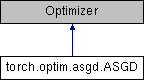Caffe2 - Python API A deep learning, cross platform ML framework
torch.optim.asgd.ASGD Class Reference
Inheritance diagram for torch.optim.asgd.ASGD:## Public Member Functions

def __init__ (self, params, lr=1e-2, lambd=1e-4, alpha=0.75, t0=1e6, weight_decay=0)

def step (self, closure=None)

## Detailed Description

```Implements Averaged Stochastic Gradient Descent.

It has been proposed in `Acceleration of stochastic approximation by
averaging`_.

Arguments:
params (iterable): iterable of parameters to optimize or dicts defining
parameter groups
lr (float, optional): learning rate (default: 1e-2)
lambd (float, optional): decay term (default: 1e-4)
alpha (float, optional): power for eta update (default: 0.75)
t0 (float, optional): point at which to start averaging (default: 1e6)
weight_decay (float, optional): weight decay (L2 penalty) (default: 0)

.. _Acceleration of stochastic approximation by averaging:
http://dl.acm.org/citation.cfm?id=131098
```

Definition at line 6 of file asgd.py.

## Member Function Documentation

 def torch.optim.asgd.ASGD.step ( self, closure = `None` )
```Performs a single optimization step.

Arguments:
closure (callable, optional): A closure that reevaluates the model
and returns the loss.
```

Definition at line 35 of file asgd.py.

The documentation for this class was generated from the following file: